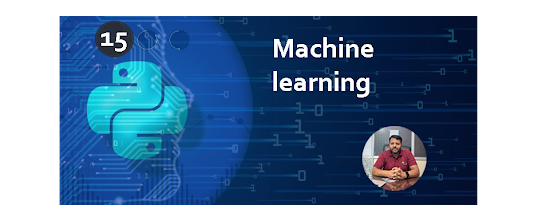0

#Why do we need Data Preprocessing?

### It involves the below steps:Getting the datasetImporting librariesImporting datasetsFinding Missing DataEncoding Categorical DataSplitting dataset into training and test setFeature scaling

from sklearn.impute import SimpleImputer
import pandas as pd
import numpy as np
from sklearn.preprocessing import LabelEncoder,OneHotEncoder
from sklearn.preprocessing import StandardScaler
from sklearn.compose import ColumnTransformer
from sklearn.model_selection import train_test_split
from sklearn.preprocessing import StandardScaler

imputer= SimpleImputer(missing_values =np.nan, strategy='mean')
#Fitting imputer object to the independent variables x.
x= data_set.iloc[:,:-1].values

imputer= imputer.fit(x[:, 1:3])
#Replacing missing data with the calculated mean value
x[:, 1:3]= imputer.transform(x[:, 1:3])
print(x)

label_encoder_x_1 = LabelEncoder()
x[: , 0] = label_encoder_x_1.fit_transform(x[:,0])
transformer = ColumnTransformer(
[('Country', OneHotEncoder(sparse=False),),],remainder='passthrough'
)
x = transformer.fit_transform(x)
print(x)
y= data_set.iloc[:,3].values
labelencoder_y= LabelEncoder()
y= labelencoder_y.fit_transform(y)
print(y)
x_train, x_test, y_train, y_test= train_test_split(x, y, test_size= 0.2, random_state=0)

#print("Training Data Set are ",x_train,y_train)

#print("Testing Data Set are",x_test,y_test)

st_x= StandardScaler()

x_train= st_x.fit_transform(x_train)

x_test= st_x.transform(x_test)

print(x_train)
print(x_test)

# Machine learning:- K-means

K-means clustering is a method for finding clusters and cluster centers in a set of unlabelled data.

cluster means group of matching data ,we can show using different label for example we can create three different sub-group for red ,green and blue to manage related data ,if item will be belonging from red color then it will be the part of red cluster.

step for clustering:-

1) prepare data using repository or from array using numpy
2)  if we want to re scale data then we can use whiten()
3)  calculate centroid point from data based on number of cluster.
4)  display possible matching from cluster with values it will return the minimum difference using 0,1 and 2 ... form
Complete code of K-means Clustering algorithm ,it will be mainly implemented in ML:-
Complete example of Clustering concept
from numpy import hstack,array
from numpy.random import rand
from scipy.cluster.vq import *
data = vstack((rand(10,3) + array([1,1,1]),rand(10,3)))
centroids,_ = kmeans(data,5)
print(centroids)
clx,_ = vq(data,centroids)
print(clx)